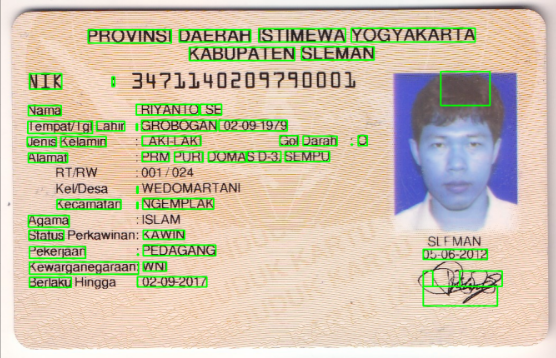# Confidence in KTP-OCR using PytesseractIn previous blog, we already learn how to crop an image https://about.lovia.id/getting-cordinate-and-cropping-an-image-with-opencv/. Then we will learn how to got confidence using pytesseract, After much searching, there was some some ways to got confidence in my KTP-OCR. Pytesseract give us a lot of syntax that can we use, such as :

```#this line of code will extract your image into string
print(pytesseract.image_to_string(Image.open('test.png')))

# Batch processing with a single file containing the list of multiple image file paths print(pytesseract.image_to_string('images.txt'))

# Get information about orientation and script detection
print(pytesseract.image_to_osd(Image.open('test.png')))```

And many others, to got the confidence, pythesseract already give line of code, it was:

`text1 = pytesseract.image_to_data(Image.open('test.png'))`

This line of code will output confidence, boxes on image, page number, line number, etc. This code give us the confidence each word not each line, so i will change it then we will got the confidence each line.

```text = text1[text1.conf != -1]
lines = text.groupby('block_num')['text'].apply(list)
conf = text.groupby(['block_num'])['conf'].mean()

print(text)
print(lines)
print(conf)```

the output would be like this:

and if u want to see the box the boxes of text on the image, just use this code:

```n_boxes = len(text1['text'])
for i in range(n_boxes):
if int(text1['conf'][i]) > 60:
(x, y, w, h) = (text1['left'][i], text1['top'][i], text1['width'][i], text1['height'][i])
img = cv2.rectangle(img, (x, y), (x + w, y + h), (0, 255, 0), 2)

plt.imshow(img)```

here some source that might help: